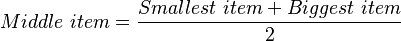# Binary search facts for kids

Kids Encyclopedia Facts

In computer science, binary search is a method used for finding an item in a sorted list. It is an algorithm that is more efficient than linear search, which will go through every item in the list of items to find a particular item. However, the given list of items must be sorted first before a binary search algorithm can be used.

The binary search algorithm works by repeatedly splitting the sorted list into two and working on the part of the list that may contain the item that you are looking for until the final list contains only one item.

## Algorithm

First, the algorithm must be given a sorted list and the item to look for, called the target value. The algorithm then compares the middle item of the sorted list with the target value. If the middle item is the same as the target value, then the position of that middle item in the sorted list is returned by the algorithm. If the middle item is larger than the target value, then the algorithm will repeat the previous step, but this time only working on the lower half of the sorted list (where all the items are smaller than the middle item). The same is done if the middle item is smaller than the target value, but the algorithm will repeat the previous step on the upper half of the sorted list (where all the items are larger than the middle item).

The position for the middle item is found using the following formula (round down to the nearest whole number):$Middle\ item = \frac{Smallest\ item + Biggest\ item}{2}$

As the algorithm will divide the sorted list into two parts, each iteration thus makes the size of the search smaller by half, which makes it very efficient when searching in a large list of items.

Here is an example of searching for the number 84 in a list of 9 numbers. The following is a table showing the 9 numbers in increasing order and their list positions:

0 1 2 3 4 5 6 7 8
3 26 35 39 47 63 73 84 95

The following is a table showing each step of the binary search algorithm. The middle item in the sorted list is in bold.

Step Sorted list of items Position of smallest item in list Position of biggest item in list Position of middle item in list
1 3, 26, 35, 39, 47, 63, 73, 84, 95 0 8 4
2 63, 73, 84, 95 5 8 6
3 84, 95 7 8 7

In this case, position 7 is returned by the algorithm after 3 steps. If the item is not inside the sorted list of items, the binary search algorithm will keep trying until the position of the smallest item is larger than the position of the biggest item before it will return as an unsuccessful search.

## Implementation

The implementation of this algorithm requires using a while loop, which will check if the position of the smallest item is larger than the position of the biggest item. Inside the loop, the algorithm will then check if the middle item is equal to, smaller than or bigger than the target value.

A possible way of writing this algorithm in Java is shown below.

public static int binarySearch(int[] array, int target) {
int smallest = 0;
int biggest = array.length - 1;

// Make sure that we still have items in the list to search in
while (smallest <= biggest) {
int middle = (smallest + biggest) / 2;

if (array[middle] == target) {
return middle;
} else if (array[middle] > target) {
biggest = middle - 1;
} else {
smallest = middle + 1;
}
}

// Return a negative number when we cannot find the target in the array
return -1;
}


## Related pagesBinary search Facts for Kids. Kiddle Encyclopedia.